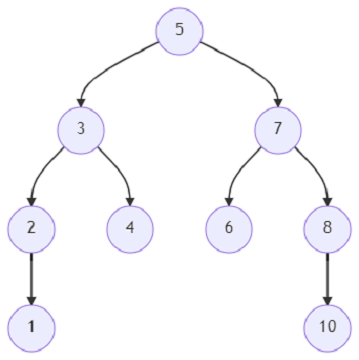# Program to find out the lowest common ancestor of a binary tree using parent pointers using Python

Suppose, we are given a binary tree and also two specific nodes x and y. We have to find out the lowest common ancestor of the two nodes from the binary tree. The lowest common ancestor in a binary tree is the lowest node of which both the nodes x and y are descendants. A particular node can also be a descendant of itself. We have to find the node and return it as an output.

The node structure of the tree is like below −

TreeNode:
data: <integer>
left: <pointer of TreeNode>
right: <pointer of TreeNode>
parent: <pointer of TreeNode>

We have to utilize the parent pointer while finding the solution.

So, if the input is likeand x = 3, y = 7; then the output will be 5.

3 and 7 are both descendants of 5, so the answer will be 5.

To solve this, we will follow these steps −

• path_p_r := a new list

• while x is not null, do

• insert x at the end of path_p_r

• x := parent of x

• while y is not null, do

• if y is present in path_p_r, then

• return y

• y := parent of y

Let us see the following implementation to get better understanding −

## Example

Live Demo

class TreeNode:
def __init__(self, data, left = None, right = None, parent = None):
self.data = data
self.left = left
self.right = right
self.parent = parent
def insert(temp,data):
que = []
que.append(temp)
while (len(que)):
temp = que
que.pop(0)
if (not temp.left):
if data is not None:
temp.left = TreeNode(data, parent=temp)
else:
temp.left = TreeNode(0,parent=temp)
break
else:
que.append(temp.left)
if (not temp.right):
if data is not None:
temp.right = TreeNode(data,parent=temp)
else:
temp.right = TreeNode(0,parent=temp)
break
else:
que.append(temp.right)
def make_tree(elements):
Tree = TreeNode(elements)
for element in elements[1:]:
insert(Tree, element)
return Tree
def search_node(root, element):
if (root == None):
return None
if (root.data == element):
return root
res1 = search_node(root.left, element)
if res1:
return res1
res2 = search_node(root.right, element)
return res2
def solve(x, y):
path_p_r = []
while x:
path_p_r.append(x)
x = x.parent
while y:
if y in path_p_r:
return y
y = y.parent
root = make_tree([5, 3, 7, 2, 4, 1, 7, 6, 8, 10])
print(solve(search_node(root, 3), search_node(root, 7)).data)

## Input

[5, 3, 7, 2, 4, 1, 7, 6, 8, 10], 3, 7

## Output

5

Updated on: 29-May-2021

87 Views